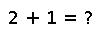# select!

select!(v, k, [by=,] [lt=,] [rev=false])

Partially sort the vector `v` in place, according to the order specified by `by`, `lt` and `rev` so that the value at index `k` (or range of adjacent values if `k` is a range) occurs at the position where it would appear if the array were fully sorted via a non-stable algorithm. If `k` is a single index, that value is returned; if `k` is a range, an array of values at those indices is returned. Note that `select!` does not fully sort the input array.

## Examples

Checking you are not a robot: